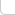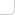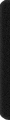# 某岛

… : "…アッカリ～ン . .. . " .. .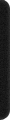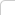April 12, 2013

## Problem A. Greg and Array

。。略）

### Analysis:

。。其实这题不需要使用线段树或是树状数组。。。
。。。对这类各种操作。。一次询问的题总有更直接的处理方法。。

。。参见咖啡枢纽。。。

## Problem C. Greg and Friends

### Brief description:

。。n 个质量分别为 1, 2 的人驾驶着一艘载重为 k 的小船渡河。
。。问把人全部送到对岸的最少往返次数。。。以及满足最少往返次数条件下的方案数。。
( n = 50）。。

### Analysis:

… 个人认为本题十分 Nice。。。很容易想到 dp[往返次数] 然后枚举  的人数这种五次方 DP。。。
。。。。比赛时总会有人误认为。。返回的时候一定只搭乘1个人才能最优。。。。
。。实际上继续观察我们会发现。。往返这两个过程。。并没有本质上的差异。。。应写成统一形式。。。。

const int N = 59;
Int f[N][N], C[N][N];
int n, k, p, q, c1, c2;

void Prep(){
swap(p, q); REP_2(a1, a2, c1+1, c2+1) f[p][a1][a2] = 0;
}

#define b (b1+b2*2)

void Roll(){
Prep(); REP_2(a1, a2, c1+1, c2+1) if (f[q][a1][a2]) REP_2(b1, b2, a1+1, a2+1) if (b &&b <= k){
f[p][c1-a1+b1][c2-a2+b2] += C[a1][b1] * C[a2][b2] * f[q][a1][a2];
}
}

int main(){

#ifndef ONLINE_JUDGE
freopen("in.txt", "r", stdin);
//freopen("out.txt", "w", stdout);
#endif

RD(n, k); k /= 50; DO(n) if (RD()==50) ++c1; else ++c2;
REP(i, n+1){C[i] = 1; REP_1(j, i) C[i][j] = C[i-1][j-1] + C[i-1][j];}

p = 0, q = 1; Prep(); f[p][c1][c2] = 1; REP(t, 2*n){

Roll();

if (f[p][c1][c2]){
printf("%d\n%d", t*2+1, int(f[p][c1][c2]));
return 0;
}

Roll();
}

printf("-1\n0");
}


## Problem D. Greg and Caves

### Brief description:

。。图案是凸的。。上下的宽度至少是 2.。。。
。。且中间存在一个梗。（可以覆盖其他所有行）。。
( n,m = 2000)

### Analysis:

。。大致也可以归类到培养皿问题。。但是因为中间有一个梗而且没有障碍。。

。。所以可以 dp 出一个方向的然后连起来。。。
。。f[][] 表示高度恰好为[]。。梗的宽度为 []。。

1 1 1 1 1 1 1
1 3 6 10 15 21 28
1 5 15 35 70 126 210
1 7 28 84 210 462 924


C(0, 0) C(1, 1) C(2, 2) C(3, 3)
C(2, 0) C(3, 1) C(4, 2) C(5, 3)
C(4, 0) C(5, 1) C(6, 2) C(7, 3)
C(6, 0) C(7, 1) C(8, 2) C(9, 3)


。。。应该可以联系挡板什么的给出个比较合理的组合解释。。。反正我是怎么都不行。。
。。然后 s[][] 表示高度至多为 []。。然后宽度为 []。。。。
。。连起来的时候用 f[][] 和 s[][] ×起来就好。

。s[][] 可以看成是浮动窗口。。从而不会引起重复计数。。。

const int N = 2009;
Int f[N][N], s[N][N];
int n, m;

int main(){

#ifndef ONLINE_JUDGE
freopen("in.txt", "r", stdin);
//freopen("out.txt", "w", stdout);
#endif

RD(n, m);

FOR_1(i, 2, m) f[i] = 1; FOR_1(i, 2, n){Int s = 0; FOR_1(j, 2, m) f[i][j] = (s += f[i-1][j]) + f[i][j-1];}
CPY(s, f); FOR_1(i, 2, n) FOR_1(j, 2, m) s[i][j] += s[i-1][j];
Int res = 0; REP_1(i, 1) FOR_1(j, 2, m) res += s[i][j] * f[n-i+1][j] * (m-j+1);

OT(res);
}


## Problem E. Yaroslav and Points

### Brief description:

。。x 轴 上分布着 n 个点。。要求支持以下两个操作。。

• 询问所有范围 [l, r] 之间的点对的距离的和。
• 移动其中一个点的坐标。

### Analysis:

… 似乎方法很多。。不过果然还是 Splay 最好弄了。。。。

/*
x
[l] [r]
*/
inline void update(){
sz = l->sz + 1 + r->sz, s = l->s + ky + r->s; // size, sum ..
ss = l->ss + (l->sz*ky-l->s) + (l->sz*r->s - r->sz*l->s) + (r->s-r->sz*ky) + r->ss; // 点对和
//      x 点和左子树。。。     //  跨越 x 点的。。        // 和右子树。。
}


。。对于询问操作而言。。。是可以直接在 splay 里面进行查找的。。但是要写成 lower_bound upper_bound 那种着实比较麻烦。。
。。而修改操作。。也需要查找某个点的位置。。而且考虑到没有重复结点。。写成前驱后继的话更不容易出错。。

（。。xwlj 的 E 题大裸数据结构要不要这样。。codeforces 果然也开始世风日下了么。。
http://codeforces.com/contest/295/submission/3515011